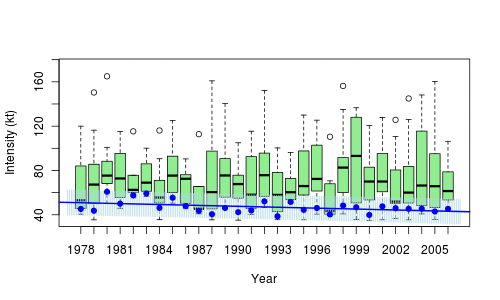# Quantile Regression (home made, part 2)

A few months ago, I posted a note with some home made codes for quantile regression… there was something odd on the output, but it was because there was a (small) mathematical problem in my equation. So since I should teach those tomorrow, let me fix them.

## Median

Consider a sample $\{y_1,\cdots,y_n\}$. To compute the median, solve$$\min_\mu \left\lbrace\sum_{i=1}^n|y_i-\mu|\right\rbrace$$which can be solved using linear programming techniques. More precisely, this problem is equivalent to$$\min_{\mu,\mathbf{a},\mathbf{b}}\left\lbrace\sum_{i=1}^na_i+b_i\right\rbrace$$with $a_i,b_i\geq 0$ and $y_i-\mu=a_i-b_i$, $\forall i=1,\cdots,n$. Heuristically, the idea is to write $y_i=\mu+\varepsilon_i$, and then define $a_i$‘s and $b_i$‘s so that $\varepsilon_i=a_i-b_i$ and $|\varepsilon_i|=a_i+b_i$, i.e. $$a_i=(\varepsilon_i)_+=\max\lbrace0,\varepsilon_i\rbrace=|\varepsilon|\cdot\boldsymbol{1}_{\varepsilon_i>0}$$and$$b_i=(-\varepsilon_i)_+=\max\lbrace0,-\varepsilon_i\rbrace=|\varepsilon|\cdot\boldsymbol{1}_{\varepsilon_i<0}$$denote respectively the positive and the negative parts.

Unfortunately (that was the error in my previous post), the expression of linear programs is$$\min_{\mathbf{z}}\left\lbrace\boldsymbol{c}^\top\mathbf{z}\right\rbrace\text{ s.t. }\boldsymbol{A}\mathbf{z}=\boldsymbol{b},\mathbf{z}\geq\boldsymbol{0}$$In the equation above, with the $a_i$‘s and $b_i$‘s, we’re not far away. Except that we have $\mu\in\mathbb{R}$, while it should be positive. So similarly, set $\mu=\mu^+-\mu^-$ where $\mu^+=(\mu)_+$ and $\mu^-=(-\mu)_+$.

Thus, let$$\mathbf{z}=\big(\mu^+;\mu^-;\boldsymbol{a},\boldsymbol{b}\big)^\top\in\mathbb{R}_+^{2n+2}$$and then write the constraint as $\boldsymbol{A}\mathbf{z}=\boldsymbol{b}$ with $\boldsymbol{b}=\boldsymbol{y}$ and $$\boldsymbol{A}=\big[\boldsymbol{1}_n;-\boldsymbol{1}_n;\mathbb{I}_n;-\mathbb{I}_n\big]$$And for the objective function$$\boldsymbol{c}=\big(\boldsymbol{0},\boldsymbol{1}_n,-\boldsymbol{1}_n\big)^\top\in\mathbb{R}_+^{2n+2}$$

To illustrate, consider a sample from a lognormal distribution,

n = 101 set.seed(1) y = rlnorm(n) median(y)  1.077415

For the optimization problem, use the matrix form, with $3n$ constraints, and $2n+1$ parameters,

So far so good…

## Quantile Regression

Consider the following dataset, with rents of flat, in a major German city, as function of the surface, the year of construction, etc.

base=read.table("http://freakonometrics.free.fr/rent98_00.txt",header=TRUE)

The linear program for the quantile regression is now$$\min_{\boldsymbol{\beta}^+,\boldsymbol{\beta}^-,\mathbf{a},\mathbf{b}}\left\lbrace\sum_{i=1}^n\tau a_i+(1-\tau)b_i\right\rbrace$$with $a_i,b_i\geq 0$ and $$y_i=\boldsymbol{x}^\top[\boldsymbol{\beta}^+-\boldsymbol{\beta}^-]+a_i-b_i$$$\forall i=1,\cdots,n$ and $\beta_j^+,\beta_j^-\geq 0$ $\forall j=0,\cdots,k$. So use here

require(lpSolve) tau = .3 n=nrow(base) X = cbind( 1, base$area) y = base$rent_euro K = ncol(X) N = nrow(X) A = cbind(X,-X,diag(N),-diag(N)) c = c(rep(0,2*ncol(X)),tau*rep(1,N),(1-tau)*rep(1,N)) b = base$rent_euro const_type = rep("=",N) r = lp("min",c,A,const_type,b) beta = r$sol[1:K] - r$sol[(1:K+K)] beta  148.946864 3.289674 Of course, we can use R function to fit that model library(quantreg) rq(rent_euro~area, tau=tau, data=base) Coefficients: (Intercept) area 148.946864 3.289674 Here again, it seems to work quite well. We can use a different probability level, of course, and get a plot plot(base$area,base$rent_euro,xlab=expression(paste("surface (",m^2,")")), ylab="rent (euros/month)",col=rgb(0,0,1,.4),cex=.5) sf=0:250 yr=r$solution[2*n+1]+r$solution[2*n+2]*sf lines(sf,yr,lwd=2,col="blue") tau = .9 r = lp("min",c,A,const_type,b) tail(r$solution,2)  121.815505 7.865536 yr=r$solution[2*n+1]+r$solution[2*n+2]*sf lines(sf,yr,lwd=2,col="blue")And we can adapt the later to multiple regressions, of course,

X = cbind(1,base$area,base$yearc) K = ncol(X) N = nrow(X) A = cbind(X,-X,diag(N),-diag(N)) c = c(rep(0,2*ncol(X)),tau*rep(1,N),(1-tau)*rep(1,N)) b = base$rent_euro const_type = rep("=",N) r = lp("min",c,A,const_type,b) beta = r$sol[1:K] - r$sol[(1:K+K)] beta  -5542.503252 3.978135 2.887234 to be compared with library(quantreg) rq(rent_euro~ area + yearc, tau=tau, data=base) Coefficients: (Intercept) area yearc -5542.503252 3.978135 2.887234 Degrees of freedom: 4571 total; 4568 residual # Talk on quantiles at the R Montreal groupThis afternoon, I will be giving a two-hour talk at McGill on quantiles, quantile regressions, confidence regions, bagplots and outliers. Before defining (properly) quantile regressions, we will mention regression on (local) quantiles, as on the graph below, on hurricanes,In order to illustrate quantile regression, consider the following natality database, base=read.table( "http://freakonometrics.free.fr/natality2005.txt", header=TRUE,sep=";") We can use it produce those nice graphs we can find in several papers, modeling weight of newborns, u=seq(.05,.95,by=.01) coefstd=function(u) summary(rq(WEIGHT~SEX+ SMOKER+WEIGHTGAIN+BIRTHRECORD+AGE+ BLACKM+ BLACKF+COLLEGE,data=base,tau=u))$coefficients[,2]
coefest=function(u) summary(rq(WEIGHT~SEX+
SMOKER+WEIGHTGAIN+BIRTHRECORD+AGE+ BLACKM+
BLACKF+COLLEGE,data=base,tau=u))\$coefficients[,1]
CS=Vectorize(coefstd)(u)
CE=Vectorize(coefest)(u)The slides can be downloaded on the blog, as well as the R-code.